# Average Velocity Formula (displacement over time)

Average Velocity Formula (displacement over time)

The velocity of an object is the rate at which it moves from one position to another. The average velocity is the difference between the starting and ending positions, divided by the difference between the starting and ending times. Velocity has a magnitude (a value) and a direction. The unit for velocity is meters per second (m/s).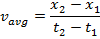vavg = average velocity (m/s)

x1 = the start position of an object (m)

x2 = the end position of an object (m)

t1 = the start time of the motion (s)

t2 = the end time of the motion(s)

Average Velocity Formula (displacement over time) Questions:

1) On a driving vacation, a boy sees a road sign that says Toronto is 220 km away. Exactly one hour later, he sees a sign that says Toronto is 100 km away. What is the velocity of the vehicle the boy is in, expressed in meters per second?

Answer: The choice of direction is important when solving velocity problems. In this case, the positions are expressed as distances away from a location. If the location, Toronto, is chosen as x = 0, and the distance values are chosen to be positive, then the vehicle is moving in the negative x direction. Using this definition for direction, the resulting value of velocity will be negative. The start position x1 = 220 km, and the end position x2 = 100 km. The travel time is given as a difference, so the start time is chosen to be t1 = 0 hours. The end time is t2 = 1.0 hours. The question asks for the velocity to be stated in meters per second, so these positions and times must be converted. There are 60 minutes in an hour, and 60 seconds in a minute, and so 1 hour is: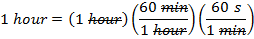1 hour = 60 x 60 s

1 hour = 3600 s

There are 1000 meters in 1 kilometer, and so 1 kilometer is:1 km = 1000 m

Using these conversions, the positions and times are: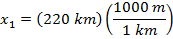∴x1 = 220 000 m∴x2 = 100 000 m∴t1 = 0 s∴t2 = 3600 s

The average velocity can be found using the formula: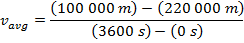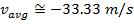The average velocity is -33.33 m/s, with the direction defined as above. This means that the average velocity can also be stated as 33.33 m/s, toward Toronto.

2) A homing pigeon was released from its cage at 10:00 am. At that time, the cage was 108.0 km from the pigeon's home. If the average velocity of the pigeon is 20.0 m/s, at what time should the pigeon arrive back at its home?

Answer: The problem is easiest to solve if the position of the cage is assigned to be x1 = 0.0 m. The pigeon's home is at position x2, which is: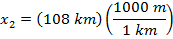∴x2 = 108 000 m

The arrival time can be found by rearranging the average velocity formula: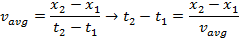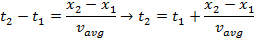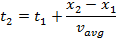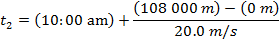t2 = (10:00 am) + (5400 s)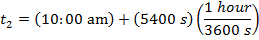t2 = (10:00 am) + 1.5 hours

∴t2 = 11:30 am

The pigeon should arrive at home at 11:30 am.

 Related Links: